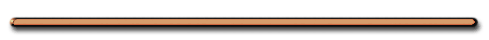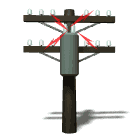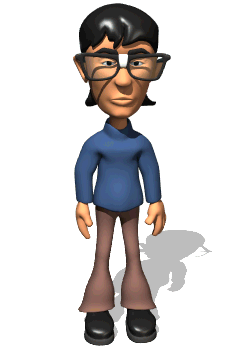"Which kind of current do we have in our homes?""Alternating Current"

Series or Parallel??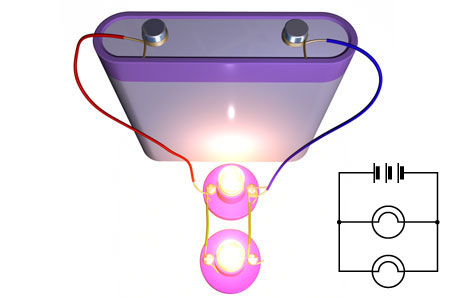Dorling Kindersley Books

Bozeman Science

2) Parallel Circuit - circuit in which each component has its own circular path back to the source.Dorling Kindersley Books

Ex 2)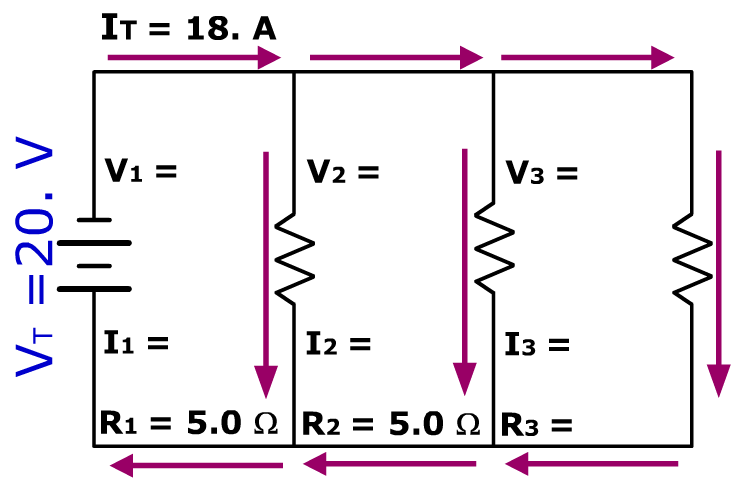a) IT = I1 + I2 + I3 + ...

For example:

If I1 = 2 amps,
I2 = 3 amps
I3 = 4 amps

(ignore picture above)

Then ...

IT = 9 amps

b) The voltage drops across each component in parallel are equal

VT  = V1 = V2 = V3

VT = Source Voltage

** Write in the correct voltagesc) V1 = I1R1

V2 = I2R2

V3 = I3R3  etc.

d) Current in 1st & 2nd resistor?

e) What’s R3?